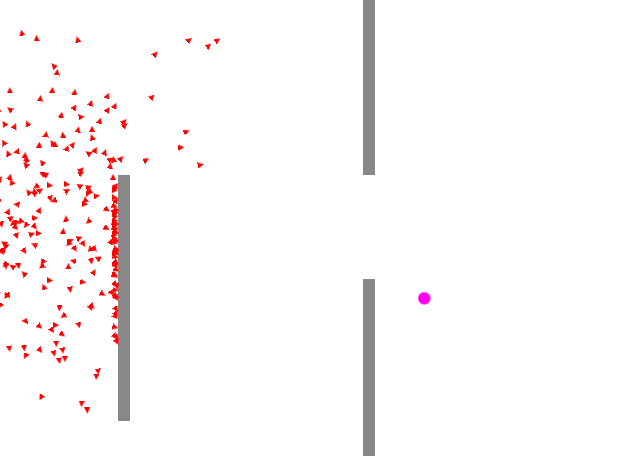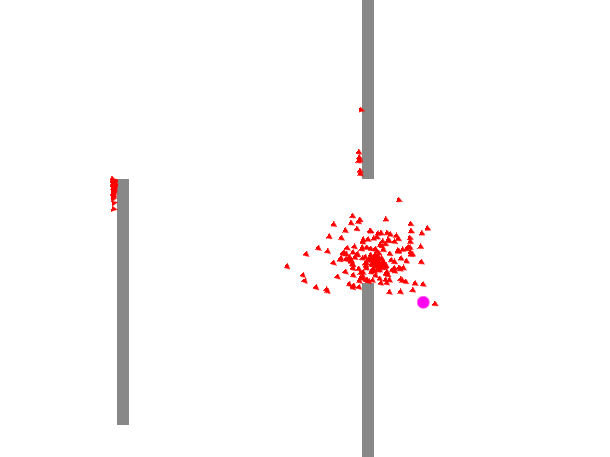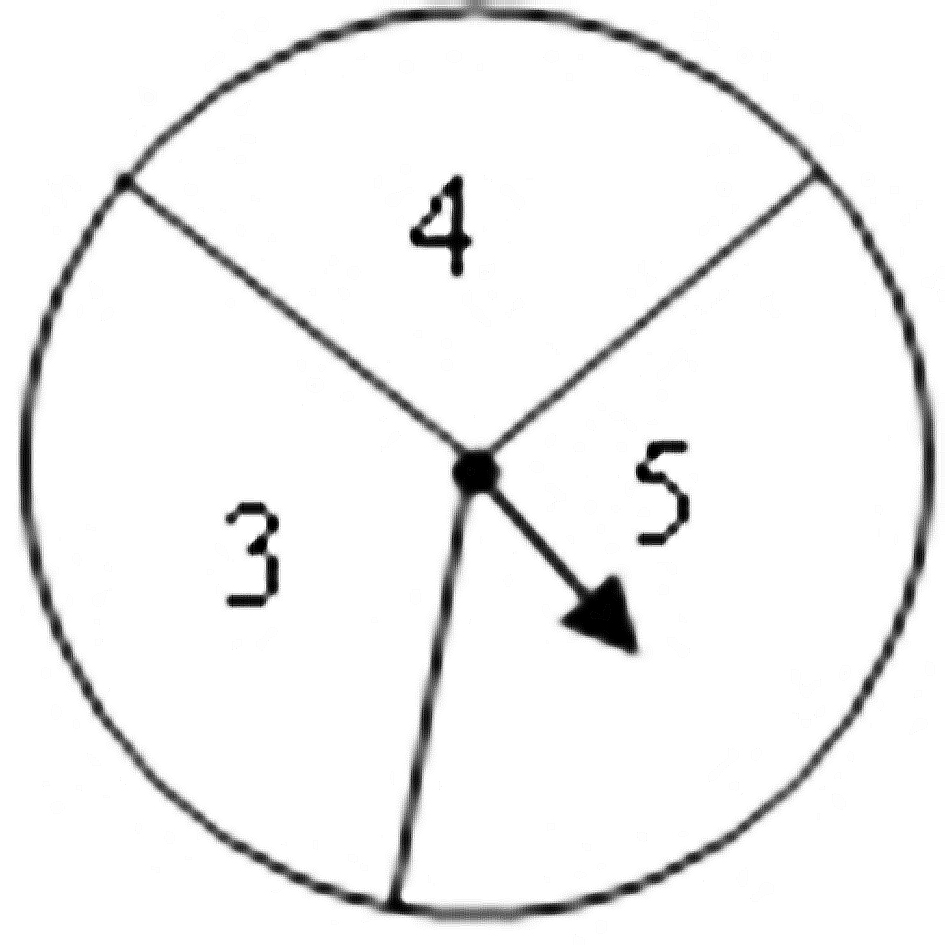# 遺傳算法-智能鳥群

## 提綱：

1.1 群體

1.2 基因型和表現型

1.3 突變

1.4 選擇

1.5 遺傳

2.1 小鳥的基因型和表現型

2.2 小鳥的基因如何影響小鳥的飛行路線

2.3 小鳥的適應性

2.4 選擇：選擇適應度更高的小鳥

2.5 突變：增加基因的豐富性

2.6 繁殖：產生新的DNA

2.7 群體的繁殖

三、總結和優化

#### 智能鳥群1. 群體

2. 基因型和表現型

3. 遺傳、突變和選擇

## 二、智能鳥群的實現

#### 1. 小鳥的基因型和表現型

 1 class DNA {
2     private _genes=[];
3     private _fitness:number;
4     private _maxforce=0.5;//最大推力為0.5
6
7     public constructor() {
9             let force=Vector2D.random2D();
10             force.mult(Math.random()*this._maxforce);//初始狀態，每一時刻的推力是隨機的。
11             this._genes.push(force);
12         }
13     }
14
15     public get genes(){
16         return this._genes;
17     }
18 } 

#### 2. 小鳥的基因如何影響小鳥的飛行路線

 1 class Bird extends egret.Sprite {
2
3     public location:Vector2D;
4     public velocity:Vector2D;
5     public acceleration:Vector2D;
6     public mass:number;
7     private shape:egret.Shape;
8
9     public target:Vector2D;
10     public dna:DNA;
11     private geneCounter=0;
12
13     public constructor(mass:number,x:number,y:number,target:Vector2D=new Vector2D(0,0)) {
14         super();
15         this.dna=new DNA();
16         this.mass=mass;
17         this.location=new Vector2D(x,y);//小鳥位置
18         this.velocity=new Vector2D(0,0);//小鳥的速度
19         this.acceleration=new Vector2D(0,0);//小鳥加速度
20         this.target=target;
21
22         this.shape=new egret.Shape();
23         let g=this.shape.graphics;
24         g.clear();
25         g.beginFill(0xff0000);
26         g.moveTo(0,0);
27         g.lineTo(-10,-5);
28         g.lineTo(-10,5);
29         g.lineTo(0,0);
31         this.shape.x=this.location.x;
32         this.shape.y=this.location.y;
33     }
34
35     public run(){
36         this.geneCounter++;
37         this.applyForce(this.dna.genes[this.geneCounter]);//將基因對應時刻的力作用在小鳥上
38         this.update();
39         this.display();
40     }
41
42     public applyForce(force:Vector2D){
43         let f:Vector2D=Vector2D.div(force,this.mass);//力除以質量為加速度
45     }
46
47     public update(){
50         this.acceleration.mult(0);
51     }
52
53     public display(){
54         this.shape.x=this.location.x;
55         this.shape.y=this.location.y;
57         this.shape.rotation=angle;
58     }
59 }

#### 3. 小鳥的適應性

1 /*適應度計算*/
2 public fitness(){
3     let d=Vector2D.dist(this.location,this.target);//計算小鳥離目標點的距離，this.target為小鳥的目標點
4     this._fitness=Math.pow(1/d,2);
5 }
6
7 public getFitness(){
8     return this._fitness;
9 }

#### 4. 選擇：選擇適應度更高的小鳥

 1 public selection(){
2     this.matingPool=[];
3     let totalFitness=0;
4     for(let i=0;i<this.totalPopulation;i++){
5         totalFitness+=this.population[i].getFitness();
6     }
7
8     for(let i=0;i<this.totalPopulation;i++){
9         let n=this.population[i].getFitness()/totalFitness*200;//適應性越大的小鳥，存入交配池中的數量就越多
10         if(n<1){
11             continue;
12         }
13         for(let j=0;j<n;j++){
14             this.matingPool.push(this.population[i]);
15         }
16     }
17 }

上面的方法，先計算所有小鳥的適應度之和，最后計算每個小鳥的適應度在所有小鳥的適應度中所占比例，然后將這個比例換算為相應的個數存入交配池。可以把這種選擇方法想象成一個輪盤，某個個體的適應度所占的比例約大，它被選中的概率就越高。#### 5. 突變：增加基因的豐富性

1 public mutate(mutationRate:number){
2     for(let i=0;i<this._genes.length;i++){
3         if(Math.random()<mutationRate){
4             let force=Vector2D.random2D();
5             force.mult(Math.random()*this._maxforce);
6             this._genes[i]=force;
7         }
8     }
9 }

1 public mutate(mutationRate:number){
2     this.dna.mutate(mutationRate);
3 }

#### 6. 繁殖：產生新的DNA

 1 public crossover(partner:DNA):DNA{
2     let child=new DNA();
3     for(let i=0;i<this._genes.length;i++){
4         let random=Math.random();
5         if(random>0.5){
6             child._genes[i]=this._genes[i];
7         }else{
8             child._genes[i]=partner._genes[i];
9         }
10     }
11     return child;
12 }

/*小鳥的繁殖*/
public crossover(b:Bird):Bird{
let bird=new Bird(1,initX,initY);//小鳥質量為1，初始位置為(initX,initY)
let dna=this.dna.crossover(b.dna);
bird.dna=dna;
return bird;
}

#### 7. 群體的繁殖

 1 public reproduction(){
2
3     for(let i=0;i<this.population.length;i++){
4         let a=Math.floor(Math.random()*this.matingPool.length);
5         let b=Math.floor(Math.random()*this.matingPool.length);
6         let partnerA=this.matingPool[a];
7         let partnerB=this.matingPool[b];
8         let child=partnerA.crossover(partnerB);
9         child.target=this.target;//為小鳥設定目標
10
11         child.mutate(this.mutationRate);
12         this.removeChild(this.population[i]);
13         this.population[i]=child;
15     }
16 }

## 三、總結和優化

1. 定義個體的基因型和表現型，基因型發生改變表現型也會隨之變化。

2. 計算群體中每個個體的適應性；

3. 選擇適應性更高的個體作為下一代的雙親(可以通過交配池實現)；

4. 通過雙親繁殖下一代，產生的下一代會發生基因突變；

5. 返回2進行下下一代的繁殖;

posted @ 2019-08-28 12:29  snsart  閱讀(...)  評論(...編輯  收藏